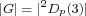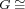Volume 32 • Number 3 • 2009
 • Quasirecognition by Prime Graph of 2Dp(3) Where p = 2n + 1 ≥ 5 is a Prime A. Babai, B. Khosravi and N. Hasani Abstract. In this paper as the main result, we show that ifis a finite group such that, where,is a prime number, thenhas a unique non-abelian composition factor isomorphic to. We also show that ifis a finite group satisfyingand, then. As a consequence of our result we give a new proof for a conjecture of W. J. Shi and J. X. Bi [A characteristic property for each finite projective special linear group, in Groups-Canberra 1989, 171-180, Lecture Notes in Math., 1456, Springer, Berlin] for. Application of this result to the problem of recognition of finite simple groups by the set of element orders are also considered. 2000 Mathematics Subject Classification: 20D05, 20D60. Full text: PDF